Course Content

Ultimate NumPy

##Multidimensional Indexing

Now that you are able to access elements in 1D arrays, it’s time to learn indexing in higher dimensional arrays.

### 2D Arrays Indexing

Let’s first have a look at a 2D array example:This is a `2x3` array which means that it consists of `2` 1D arrays which lie along axis 0, and each of these 1D arrays have `3` elements which lie along axis 1.

The images below will clarify positive and negative indexing in 2D arrays: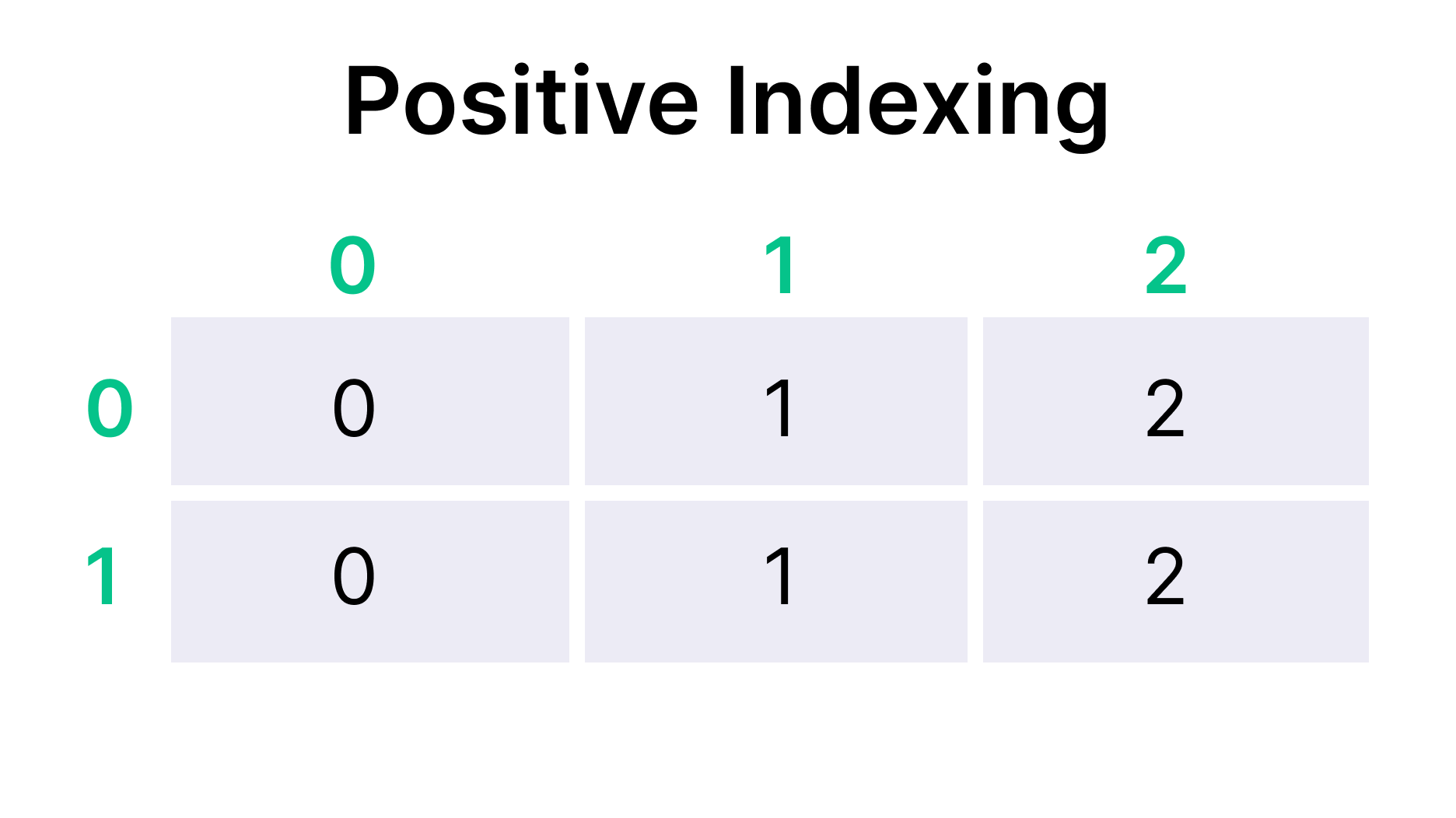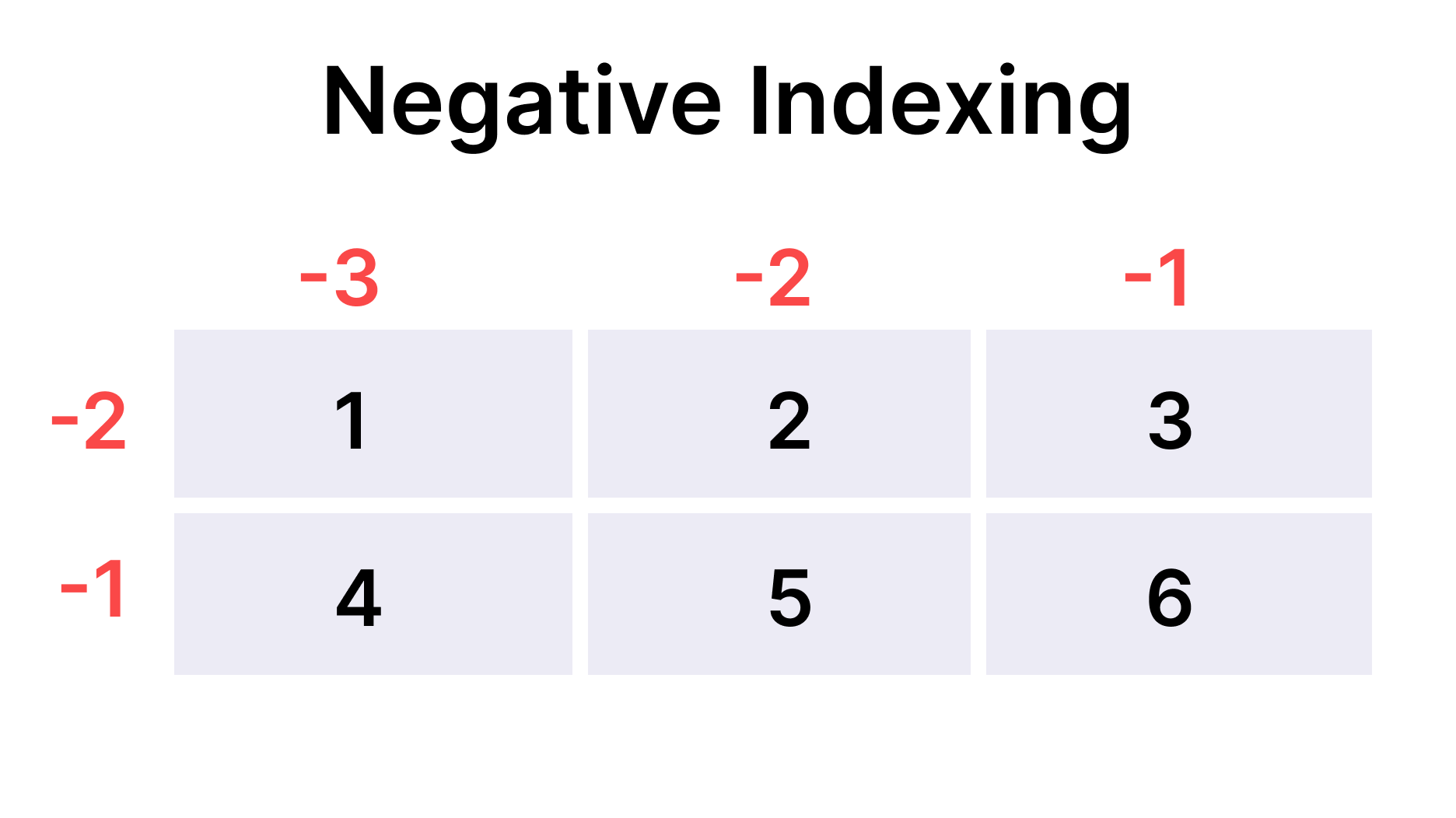As you can see, indexing along each of the axes is absolutely identical to indexing in 1D arrays.

### Accessing Elements in 2D Arrays

In 1D arrays we accessed its elements via specifying the index of this element in square brackets. If we did the same in 2D arrays, we would retrieve a 1D array at the specified index which actually may be exactly what we need.

In case we want to retrieve a particular element of an inner 1D array, however, we should specify an index of the 1D array (along axis 0) and the index of its element (along axis 1), e.g, `array[0, 1]`. We could also write `array` as we do with Python `list`, however it less efficient, since it performs the search twice for each index instead of once.

Note

Once again, if a specified index is out of bonds, then an `IndexError` is thrown, so be cautious of that.

Let’s have a look at an example:### 3D Arrays Indexing

Here is a 3D array example: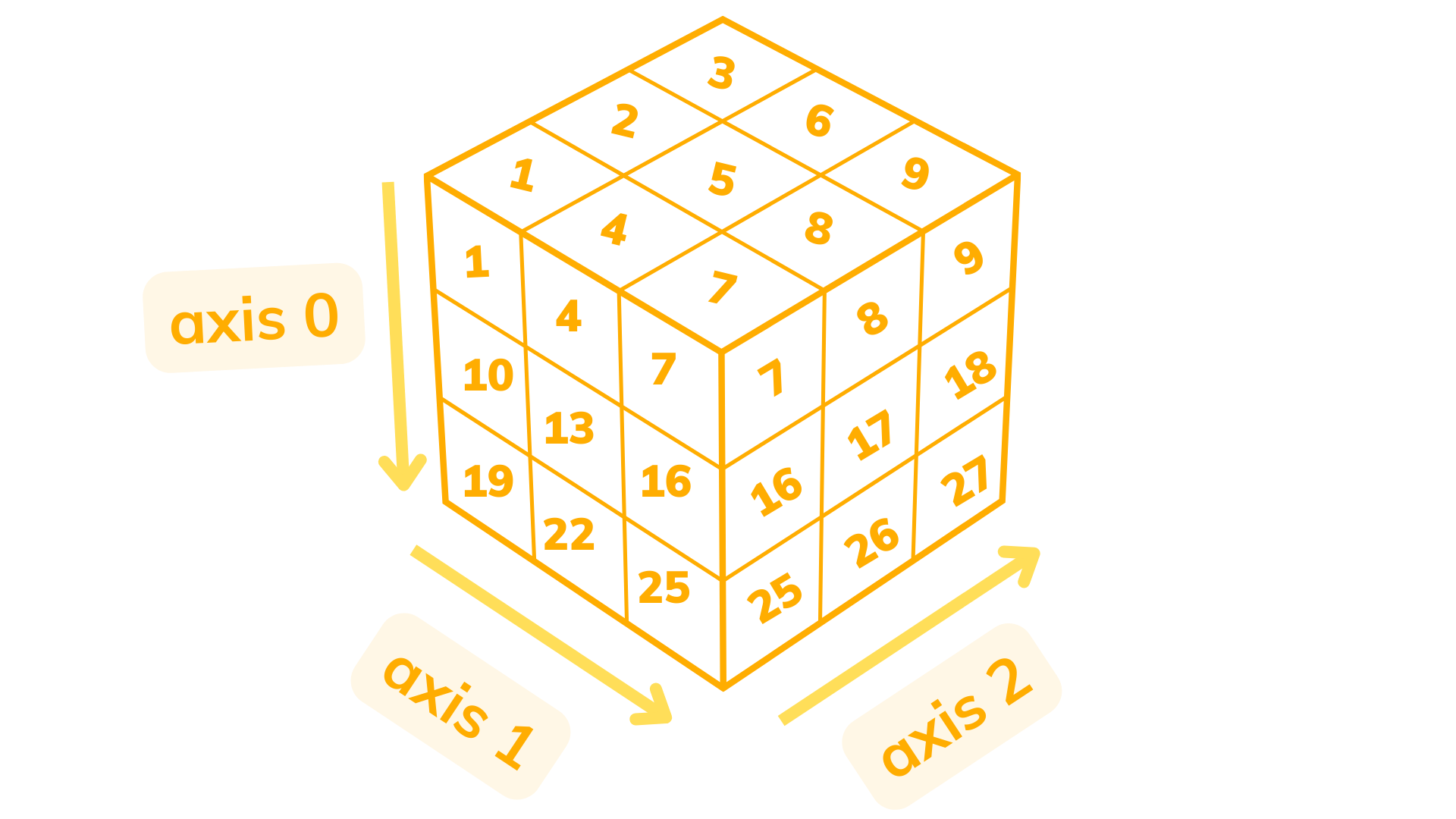This is a `3x3x3` array which means that it consists of 3 2D arrays which lie along axis 0, each of these 2D arrays consist of 3 1D arrays which lie along axis 1, and each of these 1D arrays have 3 elements lying along axis 2.

The images below will clarify positive and negative indexing in 3D arrays: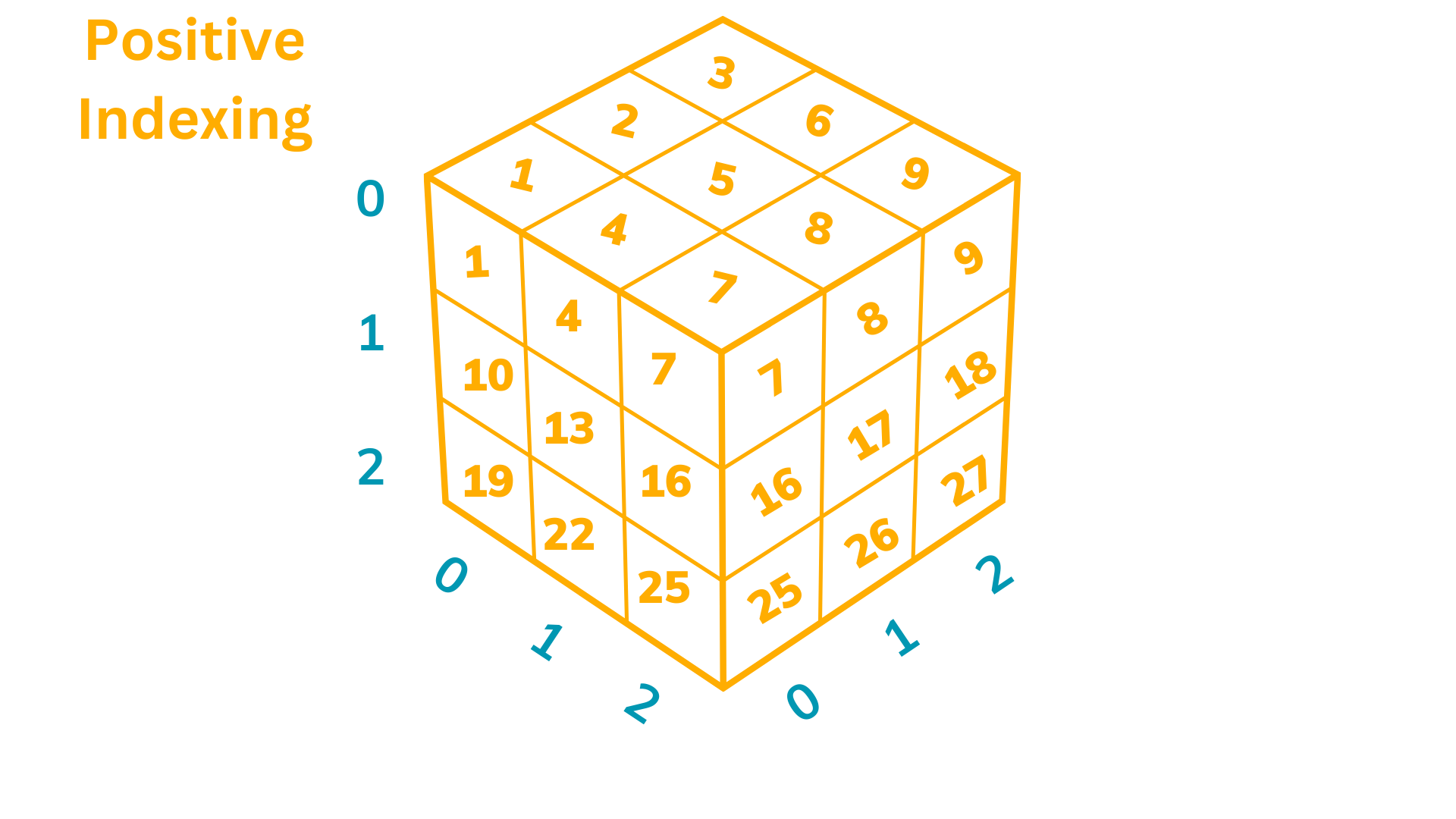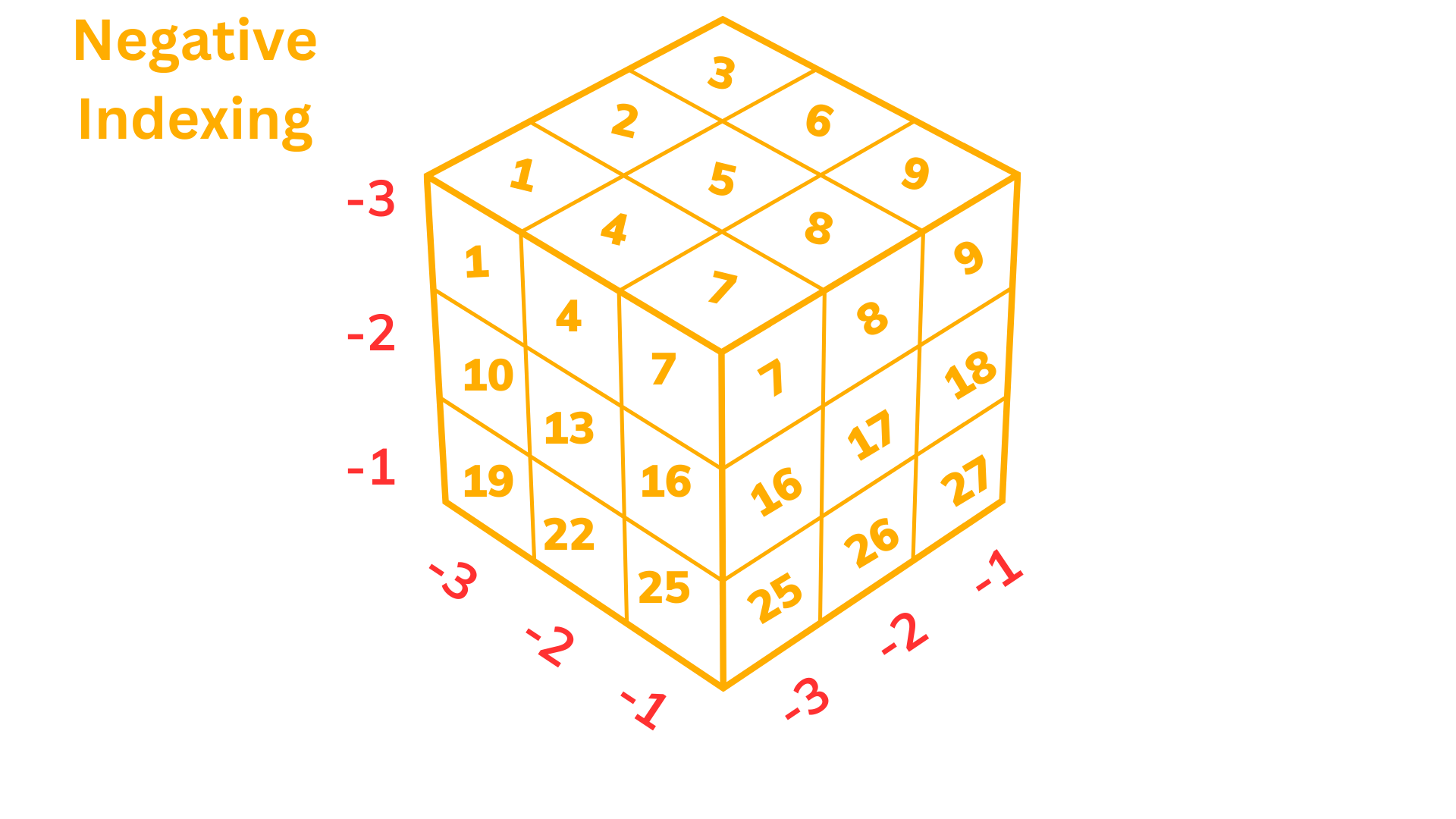### Accessing Elements in 3D Arrays

In order to access a 2D array you should specify a single index in the square brackets, e.g, `array`. If you want to access a 1D array you should specify two indices, e.g., `array[1, 0]`. In order to access a single element of a 1D array you should you use three indices, e.g., `array[1, 0, 0]`.

Here is an example:1. Use only positive indices to retrieve the top left element of the `identity_matrix` and store the result in `first_diagonal_element`.
2. Use only positive indices to retrieve the second element of the second 1D array of the `identity_matrix` and store the result in `second_diagonal_element`.
3. Use only negative indices to retrieve the bottom right element of the `identity_matrix` and store the result in `third_diagonal_element`.